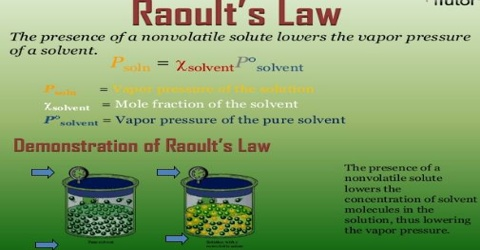# Derivation of Raoult’s Law

Derivation of Raoult’s Law

The vapor pressure of a liquid is governed by the ‘escaping tendency’ of the molecules in the liquid and the number of molecules per unit volume. If a solute is dissolved, the number of solvent molecules per unit volume decreases, since in the solution solute molecules will also be present. There is a negative deviation when the vapor pressure is lower than expected from the law. This occurs when forces between particles are stronger than those between particles in pure liquids. If we assume that the intermolecular forces between the solvent molecules remain unchanged, which is true for dilute solutions, then the number of solvent molecules leaving the unit area of the solution surface per unit time will be less than the number leaving the surface of the pure solvent under the same conditions. This action is observed in a mixture of chloroform and acetone. Here, hydrogen bonds cause the deviation. Another example of negative deviation is in a solution of hydrochloric acid and

Hence the vapor pressure of the solution will be lower than that of the solvent because the number of molecules per unit volume of the solvent in the vapor phase over liquid solvent will be more than the number of solvent molecules over the solution. The vapor pressure p of the solution will be proportional to the ratio of the number of solvent molecules, N, in solution to the total number of molecules in the solution, (n + N). Thus

p = K [N / (n + N)] = K xN

where K is the proportionality constant and xN is the mole fraction of the solvent. For the pure solvent xN = I and p become equal to p0. Therefore, K is equal to p0. Thus,

p = P0 xN.

which is one form of Raoult’s law.

It has, however, been assumed that the vapor of the solvent above the solution and the pure liquid behaves like an ideal gas. Both components of the mixture escape solution more eagerly than if the components were pure. This action is observed in mixtures of benzene and methanol and mixtures of chloroform and ethanol. Further assumptions have been made regarding the constancy of the intermolecular forces of the liquid in the presence of the solute.

Raoult’s law states: The vapor pressure of an ideal solution is dependent on the vapor pressure of each chemical component and the mole fraction of the component present in the solution.

Raoult’s law states that in a solution, the vapor pressure of a constituent at a given temperature is equivalent to the mole fraction of that constituent in the solution multiplied by the vapor pressure of that constituent in the pure state.

Understanding Raoult’s law –

Raoult’s Law gives us a quantitative relation between the fractional vapor pressure of components present in the vapor solution-phase above a liquid solution and the mole fraction of that component in the solution. Raoult’s Law is defined as a solution that has one or more unstable liquids in it.

Limitations of Raoult’s Law

• It is relevant only to perfect liquid solutions. Nevertheless, the law applies to most perfect solutions and has been a base for further studies in this field.
• The results obtained for some multifaceted, concentrated solutions that have important intermolecular forces varying significantly from the law.